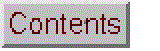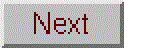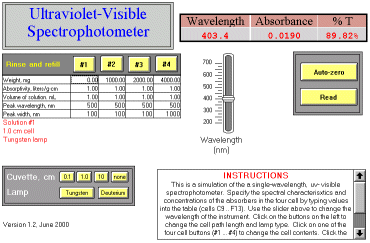# Simulation of U.V.-Vis. PhotometerVersion 1.2 (June, 2000). Click to see a larger view.

Simulation of a variable-wavelength uv-visible spectrophotometer with a 200 - 700 nm wavelength range, switchable tungsten and deuterium light sources, four interchangable quartz cuvettes, percent transmission and absorbance readout, auto-zero button, and realistic sources of error and non-linearity. Students specify sample characteristics, select wavelength, cell path length, select deuterium or tungsten lamp, and perform measurements. Realistic modeling of lamp spectral characteristics, cell transmission variations, photon and detector noise, unabsorbed stray light, and instrument non-linearity caused by finite spectral bandpass.

Version 1.2 (June, 2000) has a fixed 5 nm spectral bandpass and models a single absorber in solution. Allows the student to specify the solute weight, solution volume, and the absorptivity, peak wavelength, and peak width of the absorber in each of the four cells. Useful for reinforcing the Beer-Lambert Law and its limits and for introducing the effects of noise and non-linearity due to optical deviations from the Beer-Lambert Law.

Version 1.3 is similar to version 1.2, with the addition of a "Statistics" button that takes 30 absorbance readings automatically (without removing and replacing the cell) and calculates the mean (average), standard deviation, and percent relative standard deviation of the absorbance.

Operating Instructions:
Specify the spectral characteristics and concentrations of the absorbers in the four cell by typing values into the table (cells C9 .. F13). Use the slider above to change the wavelength of the instrument. Click on the buttons on the left to change the cell path length and lamp type. Click on one of the four cell buttons (#1 ... #4) to change the cell contents; this simulates removing the cell, replacing its contents, and replacing it in the light beam. Click the Auto-zero button to zero the instrument on the current cell contents. Click the Read button to take another reading of absorbance without disturbing the cells.

Wingz player application and basic set of simulation modules, for windows PCs or Macintosh

### Cell definitions and equations

Corrected August 13, 2000.
Inputs:

Rinse and refill table
Cell number: 1 2 3 4
Weight, mg C9 D9 E9 F9
Absorptivity, liters/g-cm C10 D10 E10 F10
Volume of solution. mL C11 D11 E11 F11
Peak wavelength, nm C12 D12 E12 F12
Peak width, nm C13 D13 E13 F13

Wavelength (cell G4), controled by the slider
path (cell M3): absorption path length in cm, set by the three
"Cuvette, cm" buttons (0.1, 1, 10).
background (cell O3, mirrored in cell R6), default value 0.03
LampInt (cell M6), set by lamp buttons (see lamp button scripts below)
LampMax (cell M7), set by lamp buttons (see lamp button scripts below)
LampWidth (cell M8), set by lamp buttons (see lamp button scripts below)
Stray light = (cell S6), default value 0.01%
Photon noise factor (cell W6) default value 0.0001
Detector noise factor (cell X6), default value 0.0004

Calculated quantities

Theoretical peak absorbance table:
Cell # analyte absorbance
1 =0.001*C9*C10*path/C11
2 =0.001*D9*D10*path/D11
3 =0.001*E9*E10*path/E11
4 =0.001*F9*F10*path/F11

Spectral characteristics table:
M O R
Lamp Analyte Background
6 height **** *** =background
7 position **** *** 150.00
8 width **** *** 1000.00

*** set by cell buttons
**** set by lamp buttons

Array calculations:

L9..L19: slice=wavelength-5
M9..M19: Lamp=M\$6*exp(-((slice-M\$7)/M\$8)^2)
N9..N19: slit width = trangular function, 5 nm half-width
O9..O19: analyte=O\$6*exp(-((slice-O\$7)/O\$8)^2)
P9..P19: Interference=P\$6*exp(-((slice-P\$7)/P\$8)^2)
Q9..Q19: reagent=Q\$6*exp(-((slice-Q\$7)/Q\$8)^2)
R9..R19: background=R\$6*exp(-((slice-R\$7)/R\$8)^2)

T9..T19: Total absorbance = path*sum(\$O9..\$Q9)+\$R9
U9..U19: Incident intensity = \$M9+\$S\$6
V9..V19: Transmitted intensity = \$S\$6+\$M9*10^(-\$T9)
W9..W19: photon incident = \$W\$6*sqrt(\$V9)*rand()
X9..X19: detector incident = \$X\$6*rand()
Y9..Y19: photon trans = \$W\$6*sqrt(\$V9)*rand()
Z9..Z19: detector trans = \$X\$6*rand()
AA9..AA19: meas. I-zero = \$U9+\$W9+\$X9
AB9..AB19: Meas. I = \$V9+\$Y9+\$Z9

Outputs:
AA20: total meas I-zero = sum(\$AA9..\$AA19)
AB20: total meas I = sum(\$AB9..\$AB19)
AB21: absorbance = log(\$AA\$20/\$AB\$20)
I4: Absorbance (zero corrected) = \$AB\$21-\$G\$24
J4: %T = \$AB\$20/\$AA\$20

Statistics table (version 1.3):
Average absorbance =avg(AC23..AC53)
Standard deviation =stdev(AC23..AC53)
Relative standard deviation =J17/J16

Button scripts:

"Rinse and refill" buttons:

#1
put 0.02+0.01*rand() into background
put "Solution #1" into b14
put C33 into O6
put C12 into O7
put C13 into O8
recalc

#2
put 0.02+0.01*rand() into background
put "Solution #2" into b14
put D33 into O6
put D12 into O7
put D13 into O8
recalc

and so on for cells 3 and 4.

"Cuvette, cm" buttons:

"0.1"
put 0.1 into path
put 0.02+0.01*rand() into background
put "0.1 cm cell" into b15
recalc

and so forth for the 1.0 and 10 cm buttons.

"none"
put 0 into path
put 0 into background
put "No cell in lightpath" into b15
recalc

Lamp buttons

Tungsten:
put 600 into LampMax
put 2 into LampInt
put 120 into LampWidth
put "Tungsten lamp" into b16
recalc

Deuterium:
put 200 into LampMax
put .5 into LampInt
put 120 into LampWidth
put "Deuterium lamp" into b16
recalc

Autozero:
recalc
put \$AB\$21 into g24